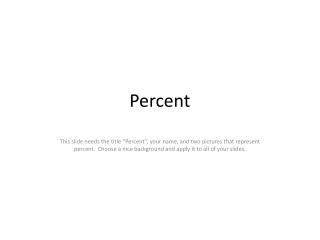DownloadDownload PresentationPercent

# Percent

Télécharger la présentation## Percent

- - - - - - - - - - - - - - - - - - - - - - - - - - - E N D - - - - - - - - - - - - - - - - - - - - - - - - - - -
##### Presentation Transcript

1. Percent This slide needs the title “Percent”, your name, and two pictures that represent percent. Choose a nice background and apply it to all of your slides.

2. Percent means “out of 100” • This slide needs the title which is above. You then must place an example like I have placed below. You must use a number other than the one I used. • 28% is the same as the fraction 28/100 • To convert this fraction to a decimal I divide 28÷100 on my calculator. The answer is 0.28. Because I am dividing by 100 the rule is “I move the decimal two places to the left”. • Solve the following using the calculator on your computer or using the rule I just stated • 1. 36÷100 • 2. 97÷100 • 3. 219÷100

3. What if the fraction does not have 100 as the denominator? • Instructions: type out the following rules • Sometimes you will get a fraction that does not have 100 as the denominator. For example you may get a mark of 27/39 on a test. It is easy to calculate this as a percentage. Follow these steps. • 1. on your calculator 27÷39x100 • This pattern will work for any fraction. I also know the rule for multiplying by 100, you move the decimal two places to the right. • Turn these fractions into percentages using the calculator on your computer. Use the exact same steps I used above. • 39/67 • 10/57 • 94/125

4. Percentages in symbols and pictures • Instructions: On this slide students are to locate three pictures from the internet, or create three pictures that show different percentages. For example, the picture below shows 25%. Students must place their pictures on this slide and what percent the picture represents. • 25%=======

5. Percent of a number • Instructions…type out the rules below • 1. You take the percent and divide it by 100 • 2. The word of means to multiply • 3. You multiply your answer from #1 by the number in the question.

6. Percent of a Number • On this slide students need to show an example of how to find the percent of a number. Follow the directions from the last slide. Here is one example. • What is 12% of 25 • 12÷100x25=3

7. GST • On this slide students must explain what GST is (what percent?) and show how to calculate the GST on an item from the store (HINT…refer to the instructions for percent of a number).

8. 10% • On this slide the students must write out the simple rule for finding 10% of any number. • To find 10% of a number you move the decimal one place to the left. This is because you are basically dividing the number by 10.

9. 10% to help you find GST • On this slide students must explain how you can use 10% of a number to help you estimate GST. They need to figure this out themselves. Most students will not get this far. Those that do should be able to do this with minimal help as we have talked about this lots in class.

10. Test Question • On this slide students are to come up with a CREATIVE, and DIFFICULT multiple choice question that has to do with percentages. The question must be answered by a grade seven student.

11. I have mastered… • On this slide students are to list three things they are now experts at when it comes to percents.

12. I wonder… • On this slide students are to write down one question they still don’t know how to answer or they are to ask a really tough question about percentages. I will answer these questions during class. Challenge the students to Google a really hard question or come up with their own. The question must be based on percentages.